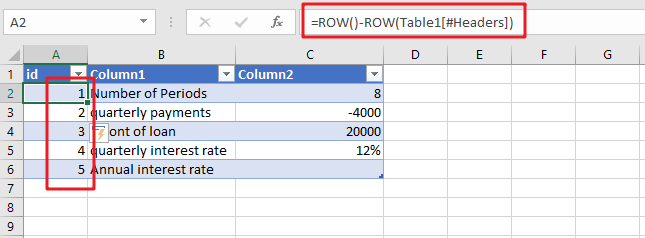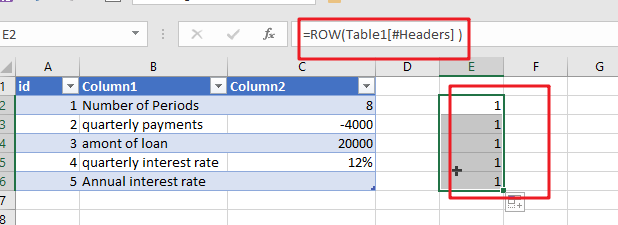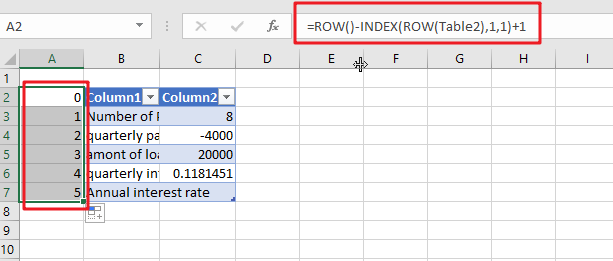# Creating a Table with Automatic Row NumberingWhen creating an Excel Table, you may use a calculation relying on the ROW function to insert row numbers automatically. The formula in A2, which has been copied down, is as follows:

`=ROW()-ROW(Table1[#Headers])`

Note: the default table name is Table1. And #Headers is the header in the Table1.

## Let’s See How This Formula Works:

When there is no parameter, the ROW function will return the row number for the current row. If A cell reference is supplied, and ROW function will retrieve the number of rows of the selected cell in question. When a range is specified, ROW returns the row number corresponding to the first row in the range.

For the above formula, there is no argument supplied to ROW, the first ROW function returns 2, located in cell A2. The second ROW function makes use of a structured reference, as follows:

`=ROW(Table1[#Headers])`The formula above works well as long as there is a header row in the table; however, the formula will fail if the heading row is disabled. If you are dealing with a table that does not have a header row, you may make use of the following method:

`=ROW()-INDEX(ROW(Table2),1,1)+1`

As in the previous formula, the initial ROW function will return the row that is currently being shown. Using the INDEX function, the first cell in the range Table2 (cell B2) is passed on to the second-ROW feature, which always returns the number 5. The following is how the formula works for the first three rows of the table:Regardless of whether or not the header row is enabled, this equation will continue to operate correctly.

### Related Functions

• Excel INDEX function
The Excel INDEX function returns a value from a table based on the index (row number and column number)The INDEX function is a build-in function in Microsoft Excel and it is categorized as a Lookup and Reference Function.The syntax of the INDEX function is as below:= INDEX (array, row_num,[column_num])…
• Excel ROW function
The Excel ROW function returns the row number of a cell reference.The ROW function is a build-in function in Microsoft Excel and it is categorized as a Lookup and Reference Function.The syntax of the ROW function is as below:= ROW ([reference])….
Related Posts

Get Address of First Cell in Range

We will learn how to get address of first cell in range in this post. At Instance, we must determine the address of first cell in range to use as the data table's value. For the DATA table, we have ...

Abbreviate Names Or Words in Excel

As an MS Excel user, you might have come across a task where you need to abbreviate different names or words, and there are also possibilities that you might have done this task manually by assuming that there isn't any ...

Filter or Extract with a Partial Match

Assume you have a table consisting of a few cells having few values, and you want to filter out the set of records with the partial match. You might take it easy and would prefer to manually filter out the ...

Extract matching values From Two Lists

Suppose that you are working with two lists containing few values, and you want to extract the matching values from those two lists into another separate list. You might prefer to manually extract the matching values from the two lists, ...

Extract all Partial Matches

Just assume that you have a range of data that contains a text string and you want to extract the few partial matches into another separate range of cells; then you might think that it's not a big deal; because ...

Extract Multiple Match Values into Separate Columns

If you have a few values/items in the excel sheet and you are thinking that with the aid of the “VlOOKUP” function you can look for a specific value, extract it and then put the matching item into the separate ...

Average the Last N Numeric Values in Excel

AVERAGE function is one of the most popular functions in Excel. Apply AVERAGE together with some other functions, we can calculate average simply for some complex situations. In this article, we will introduce you to calculate average of the last ...

How To Sum the Largest N Values in Excel

Sometimes we may want to sum the largest N numbers or top N numbers in a range. In this article, we will show you the method of “SUM the Largest N Numbers” by a simple formula which consist of SUMPRODUCT ...

How to Sum the Smallest N Values in Excel

Sometimes we may want to sum the first smallest N numbers in a range in Excel. In this article, we will show you the method of “SUM the Smallest N Numbers” by a simple formula which consist of SUMPRODUCT and ...

How to Auto Fill Weekdays or Weekends in Excel

Sometimes we may want to enter a sequence of days in excel for some purpose, and we can press Ctrl+; to insert current date into cell, and then drag the cell down to attach following days into other cells, then ...

Sidebar